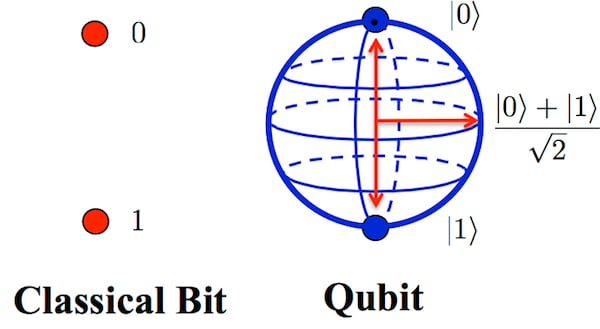# Quantum Computing ELI5 – How does Quantum Computing Work?

We’ve all heard of  “quantum computing”, but don’t you wish to understand how they work and what they do? Truth be told, the way they work is very complex, but I will explain them using common examples, so you can get a basic understanding of quantum computing.

### Normal Computers

To understand how a quantum computer works, we first need to understand how a normal computer works: in a normal computer, we use bits, which are either 0 or 1. These are transmitted through electric impulses, which are really really fast, especially in the CPU. But how does a processor use impulses or bits in order to process information? They are just 0s and 1s! CPUs use logic gates. The processor from your computer is full of these logic gates! The processor inputs 2 bits into a gate. The gate then processes the 2 bits using a rule, which depends on the type of gate. It then outputs a single bit.

There are 2 types of (basic) logic gates:

1. AND gate – An AND gate wil check if both of the bits are 1. If both of the bits inputted are 1, it will output 1 and 0 otherwise.
2. OR gate – An OR gate will check if one of the bits are 1.  If both of the bits inputted are 0, it will output 0, and 1 otherwise.Credit: openmymind.net

### Quantum Computers

There are no bits in a quantum computer, only qubits. These are not very different from normal computer bits. A qubit is not transmitted using electric impulses, they use electrons to store information. This may seem like a hard concept, but it’s not: If the electron does not exert any energy, and remains parallel to the magnetic field, it stores the value 0. If it exerts energy and it’s rotation is opposite to the magnetic field, it will store the value 1. Here’s a simple example using a kitchen timer so you can understand this concept:Let’s say the above image of a kitchen timer is our electron or qubit. This shows the value 0 because it hasn’t been wound up yet. This “electron” stores the value 0. If we wind up the timer up to 30 seconds, we have applied a force to it, and it’s rotation is now opposite to the one where it showed the value 0. This electron now shows the value 30, therefore it is opposite to the magnetic filed, storing the value 1. So a qubit can either be 1 or 0, just like a normal bit, but it can also be something else.

### Quantum superposition

Things will get a little more complicated here: As I said earlier, a qubit can be either 0 or 1, but it can also be something else: 0 and 1 in the same time! A qubit (electron) can enter a superposition: The electron starts rotating on the x and y axis. When the electron was in a continuous rotation, there was no way to tell if it was 0 or 1, because it wasn’t either of them.Credit: University of Strathclyde

When you read a qubit which is in a superposition, it will round it up. If the rotation axis is closer to the electron’s value of 1, it will be read as 1. The same goes for 0. This means that if you read a qubit in a superposition, there will be a 50% chance of it being 1, and 50% chance of it being 0. IBM have a research program where they allow anyone to make simple tests on quantum computers. You can check it out here. Using this tool, I introduced a qubit in a superposition and then read it. These were the results after 20 tries:

53.4% – 0

46.6% – 1

As we can see, there is an approximate 50/50 chance of the qubit being 1 or 0. They are not exactly 50/50 because the electron is rotating chaotically, therefore we cannot predict it, just in case of quantum entanglement, which is a much more complex phenomenon which makes qubits depend on each other. Because it is so complex, I will not describe it in this article.

Imagine you have 4 coins, and 1 is equal to HEADS and 0 is equal to TAILS. In a normal computer, if we had the set of bits 1101, the coins would be HEADS – HEADS – TAILS – HEADS, and they will never change. If we had the same set of bits (1101) but in qubits this time, they will still be HEADS – HEADS – TAILS – HEADS at first, but when they enter a superposition, is like we flip all the coins. When the coins hit the floor, the computer reads the qubits, which obviously have a high chance of NOT BEING 1101. At first, the set of bits was 1101, and now it can be 0000, 0001, 0010, 0011, 0100, 0101, 0110, 0111, 1000, 1001, 1010, 1011, 1100, 1101, 1110 or 1111. We lost all of the information.